# Python Exception Handling

## Introduction

Today I will take a new part of python for my tutorial series. In this part, you will learn  Exception Handling.

Python language has various types of Exception Handling. I will tell you some exception handling in Python and their examples.

Example of Exceptions:
1. Num1=5
2. Num2=0;
3. print(Num1/Num2)
Output: ZeroDivisionError: division by zero,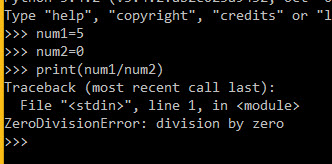1. print(“10”+6
Output: TypeError: Can't convert 'int' object to str implicitly.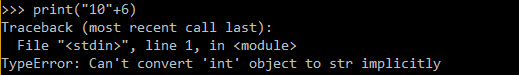1. print(2+4*num)

Output: NameError: name 'num' is not defined.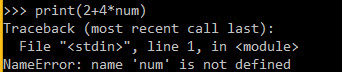1. import math
2.
3. math.exp(10000
Output: OverflowError: math range error,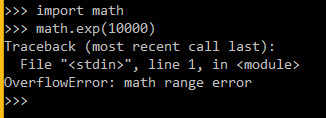1. from foo import too
Output : ImportError: No module named 'foo'.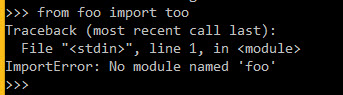1. array={'a':'1','b':'2'}
2.
3. array['c'
Output : KeyError: 'c'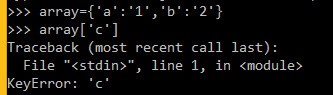1. array = [1,2,3,4,5,6,7]
2.
3. array[10
Output : IndexError: list index out of range.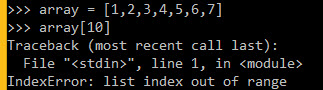So above all examples shows the exceptions in python programs with different-2 types.

So now I will tell you how to handle Exceptions

Example of Exception Handling:

try:
1. num1=5
2. num2=0
3. print(num1/num2)
4. except ZeroDivisionError:
5. print("Divided By Zero")
Output : Divided by Zero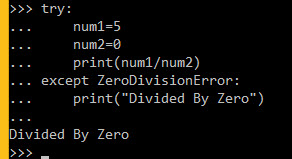try:
1. print("10"+6)
2. except TypeError:
3. print("Error occured in \"10\"+6")
Output : Error occured in "10"+6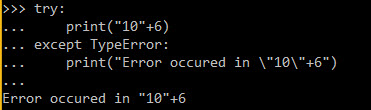try:
1. print(2+4*num)
2. except NameError:
3. print("num is not define")
Output : num is not define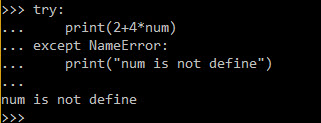So many exception handlings can handle the above Examples.

Raising Exceptions:

The raise statement forces an indication of specified Exception to occur.

try:
1. num1=5
2. num2=0
3. print(num1/num2)
4. except ZeroDivisionError:
5. raise ZeroDivisionError("Error Occured")
Output : Shown in below image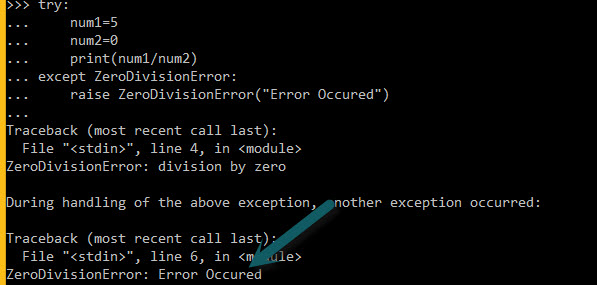So the above example, Shows a raise statement and prints the error forcibly in except module.

Finally :

In any exception occurred in code finally statement always runs after the execution of the code in the try block

try:
1. num1=5
2. num2=0
3. print(num1/num2)
4. except ZeroDivisionError:
5. print("Error Occured")
6. finally:
7. print("This code will run always")
Output: Error Occured

This code will run always.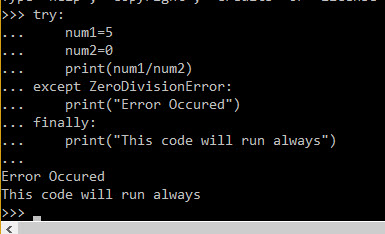So we can use the final statement in each exception for running always code in the final statement. I hope you will understand this part of the python programming language.
Next » File Handling In Python